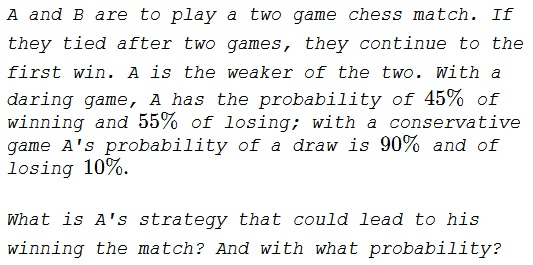# Right Strategy for a Weaker Player

### Problem### Solution 1

The strategy is to play a daring game when tied or losing and a conservative game when ahead in the match.

After two games there are four possible outcomes (from A's perspective):

$\begin{array}{c|c|c} \text{ result }&\text{ conclusion }&\text{ probability }\\ \hline LL&\text{ match lost }&0.55\cdot 0.55=0.3025\\ LW&\text{ tie-breaker required }& 0.55\cdot 0.45=0.2475\\ WL&\text{ tie-breaker required }& 0.45\cdot 0.10=0.0450\\ WD&\text{ match won }&0.45\cdot 0.90=0.4050 \end{array}$

The tie-breaker happens with the probability of $0.2925$ so A is going to play a daring game, winning with the probability of $0.45,$ making the total probability of his winning the match equal to

$0.4050+0.45\cdot 0.2925=0.536625\approx 54\%.$

### Solution 2

The approach is to use a simplified form of stochastic dynamic programming, given that we are initially facing a 2 period decision tree.

We have two regimes indexed by 1 (aggressive), 2 (conservatives) and their corresponding probabilities:

$P_1=\left( \begin{array}{c} p_{w_1} \\ p_{d_1} \\ p_{l_1} \\ \end{array} \right)$ $P_2=\left( \begin{array}{c} p_{w_2} \\ p_{d_2} \\ p_{l_2} \\ \end{array}\right)$

and a payoff vector per period for win, draw, or lose of $\lambda=\left(1,0,-1\right)$

We look at the expectation at every step. Note that for the dot product, $P_1.(1+\lambda)$ can have for notation $P_1.(1+\lambda^T)$.

$\max\left( \begin{array}{l} p_{w_1}. P_1.(1+\lambda) (\text{ aggressive, win, then aggressive})\\ p_{w_1}. P_2.(1+\lambda)(\text{ aggressive, win, then conservative})\\ \\ p_{d_1}. P_1.(\lambda)(\text{ aggressive, draw then aggressive})\\ p_{d_1}. P_2.(\lambda)(\text{ aggressive, draw then conservative})\\ \\ p_{l_1}. P_1.(-1+\lambda)(\text{ aggressive, loss, then aggressive})\\ p_{l_1}. P_2.(-1+\lambda)(\text{ aggressive, loss, then conservative})\\ \\ p_{w_2}. P_1.(1+\lambda) (\text{ conservative, win, then aggressive})\\ p_{w_3}. P_2.(1+\lambda) (\text{ conservative, win, then conservative})\\ \\ p_{d_2}. P_1.(\lambda)\\ p_{d_2}. P_2.(\lambda)\\ \\ p_{l_2}. P_1.(-1+\lambda)\\ p_{l_2}. P_2.(-1+\lambda)\\ \end{array} \right)$

### Acknowledgment

The problem and the solution are from J. G. McLoughlin et all, Jim Totten's Problems of the Week, World Scientific, 2013, problem #316. Solution 2 is by N. N. Taleb.

Jakub Šimek made this remark: One should not underestimate the ability to draw under all circumstances. Also, the oponent style makes the strategy possible, she cannot play to draw or something... Really interesting...

The problem is a very specific case of discussed by Kyle Siegrist in How to Gamble If You Must which relates to the book How to Gamble If You Must: Inequalities for Stochastic Processes by Lester E. Dubins and Leonard J. Savage.